# Comparative Analysis MHD Bioconvective Flow of Micropolar Nanofluid in Porous Medium

Comparative Analysis MHD Bioconvective Flow of Micropolar Nanofluid in Porous Medium
Department of Mathematics, University of Swaziland, Manzini M201, Swaziland

Corresponding Author Email:
msayano@uniswa.sz
Page:
727-736
|
DOI:
https://doi.org/10.18280/ijht.390306
19 August 2020
|
Accepted:
22 May 2021
|
Published:
30 June 2021
| Citation

OPEN ACCESS

Abstract:

The present study dealt with heat and mass transfer rate, and motile micro-organisms for convective micropolar nanofluid flow in a porous medium. The nanofluid contains the influence of both Brownian motion and thermodiffusion (thermophoresis). The problem considered finds applications in the areas of engineering such as reducing greenhouse effects and in the pharmaceutical applications enhancing mixing and slowing down settling particles can be mentioned. The numerical results are obtained and discussed. The results obtained suggest that micropolar fluids enhance the flow and decrease the temperature, nanoparticles concentration and gyrotactic microorganisms concentration profiles compared to viscous fluid. It is also noted that Hall parameter increases skin friction, the heat transfer rate, the mass transfer rate and density of the motile microorganisms.

Keywords:

gyrotactic microorganisms, hall current effect, radiation, nanofluid, porous medium, spectral method

1. Introduction

Studying heat and mass transfer phenomenon at small scales level attracted many researchers because of its application. To enhance the heat transfer is one of the areas of research in thermal science. Among the various techniques enhancing heat transfer in a flow adding nano size particle in the base fluid presented by Choi and Eastman  is found to be efficient thermal conductivities. The base fluid such as water, engine oil, ethylene etc. have low thermal conductivity therefore, nanofluids with the metals in it will enhance the thermal conductivity of such fluids. Nanofluids appear to behave more like single phase fluid than a solid-liquid mixture. There are various applications of nanofluid cellphones and laptops related to energy resources can be considered as an example. By developing a mathematical model Buongiorno  made a comprehensive survey of nanofluid transport mechanisms. A number of researchers studied saturating nanofluid is well documented by researchers [3-8] to mention a few.

Bioconvection happens due to a convection driven by the collective motion caused by density gradient created by collective swimming of motile microorganisms . Bioconvection nanofluid describes the impulsive pattern formation of instability due to the density stratification. Presence of microorganisms in nanofluid boost mass transfer especially in micro-volume. The motion of micro-organisms is self-intended whereas that of nanoparticle is due to random movement (i.e. Brownian motion) and thermophoretic effect in nanofluid. Bioconvection i.e. contemporaneous interplay of nanoparticles, buoyancy forces and self-propelled microorganisms find application in field of biomedical systems, bioconjugates, design of bio cells, bio microsystem, reduction of blood loos during surgeries . After Platt  introduced bioconvection and reported the physics of the curious streaming several researchers studied bioconvections flows. Nanoparticles and gyrotactic microorganisms for second grade fluid in a porous medium discussed by Khan et al.  reported microorganism concentration decreases with increase in porosity parameter. Second-grade nanofluid fluid flow over stretching sheet in the presence of gyrotactic microorganism examined by Waqas et al.  disclosed bioconvection Rayleigh number decreases velocity. Unsteady flow of Oldroyd B nanoparticles flow over a moving oscillatory surface considering gyrotatic microorganisms studied by Khan et al.  showed thermophoresis increases with Motile microorganism. Many authors studied bioconvection flow see for example [14-18].

The non-Newtonian fluid models with microstructures such as polymeric additives, colloidal suspensions and non-symmetric stress tensor is classified as micropolar fluid. After the pioneer work of Eringen  convective flow of micriopolar fluid analyzed by many authors. Ariman et al. [20, 21] reviewed micropolar fluids and showed applications in technology.

Heat transfer of nanofluid in a porous medium improve the convection heat transfer and it finds industrial applications. From applications of porous medium flow solar collectors, geothermal operations, petroleum, porous burners, can be mentioned. Fluid flow modelling through a Darcy and/or non-Darcy porous medium studied by various researchers [22-27] to mention a few. A review and applications are documented in monographs by Ingham and Pop and Ingham , Nield and Bejan .

The present paper addresses the gap that are identified in the researches mentioned above and finds applications in biotechnology, biomedical, oil refinery and bio-microsystems. The above-mentioned papers and exhaustive literature survey reveal that the study comprising radiation, magnetohydrodynamics (hall effects), the interaction of moltile microorganisms on bioconvective micropolar nanofluid flow in a porous medium has not been carried out so far. We have extended the earlier work of Zuhra et al.  for micropolar fluid by considering hall effects, porosity and thermal radiation on fluid flow, for viscous and micropolar fluid then compared. We hope the research will contribute in the magnetohydrodynamics theory it’s on part for the combined effects of the mentioned parameters. The governing partial differential equations of the modeled problem applying similarity transformations non-dimensional system of differential equations determined then solved numerically using spectral quasi linearization method (SQLM). The effects of parameters of interest are studied.

2. Modelling the Problem

Consider the two-dimensional steady, incompressible, laminar, micropolar nanofluids along with gyrotactic micro-organisms over a vertical plate in a porous medium. It is assumed transverse magnetic field of strength B0 is applied. In order microorganisms not to be absorbed the pore size is assumed to be large in the porous medium also the Brinkman-Darcy model is used. The microorganisms give rise to stabilize the nanoparticles. In order microorganisms and gyrotactic behavior not be killed it is assumed the temperature variation weak. Kuznetsov  suggested lower temperature gradient will not significantly change gyrotactic behavior. As it is reported by Sarkar et al.  from their experimental results microorganisms can withstand temperatures of 42℃. The Brownian type diffusion and thermophoretic mechanisms along with Newtonian heating are also considered. It is further assumed cross flow is caused due to the magnetic field and Hall current.

Under the above assumptions following [11, 12, 14, 32] the equations governing the flow are:

$\frac{\partial u}{\partial x}+\frac{\partial v}{\partial y}=0$                (1)

$u \frac{\partial u}{\partial x}+v \frac{\partial u}{\partial y}=\left(\frac{\mu_{f}+\kappa_{f}}{\rho_{f}}\right) \frac{\partial^{2} u}{\partial y^{2}}-\frac{\kappa_{f}}{\rho_{f}} \frac{\partial \Gamma}{\partial y}-\frac{v_{f}}{k_{1}} u-\frac{\sigma B_{0}^{2}}{\rho_{f\left(1+m^{2}\right)}}(u+m w)+\frac{g^{*}}{\rho_{f}}\left[\left(1-C_{f}\right) \beta_{T}\left(T-T_{\infty}\right)+\right. \left.\left(\rho_{p}-\rho_{f}\right)\left(C-C_{\infty}\right)+\gamma_{w}\left(N-N_{\infty}\right)\left(\rho_{m}-\rho_{f}\right)\right]$                  (2)

$u \frac{\partial w}{\partial x}+v \frac{\partial w}{\partial y}=\left(\frac{\mu_{f}+\kappa_{f}}{\rho_{f}}\right) \frac{\partial^{2} w}{\partial y^{2}}-\frac{\kappa_{j}}{\rho_{f}} \frac{\partial \Gamma}{\partial y}-\frac{v_{f}}{k_{1}} w+\frac{\sigma B_{0}^{2}}{\rho_{f}\left(1+m^{2}\right)}(u-m w)$                 (3)

$u \frac{\partial \Gamma}{\partial x}+v \frac{\partial \Gamma}{\partial y}=\gamma_{f} \frac{\partial^{2} \Gamma}{\partial y^{2}}-2 \kappa_{f} \Gamma-\kappa_{f} \frac{\partial u}{\partial y}$                (4)

$u \frac{\partial T}{\partial x}+v \frac{\partial T}{\partial y}=\alpha_{f} \frac{\partial^{2} T}{\partial y^{2}}+\frac{\sigma B_{0}^{2}}{\rho_{f}\left(1+m^{2}\right)}\left(u^{2}+w^{2}\right) +\frac{16 \sigma^{*} T_{\infty}^{3}}{3 k^{*} \rho_{f} C_{p}} \frac{\partial^{2} T}{\partial y^{2}}+\frac{(\rho c)_{p}}{(\rho c)_{f}}\left[\frac{D_{T}}{T_{\infty}}\left(\frac{\partial T}{\partial y}\right)^{2}\right. \left.+D_{B}\left(\frac{\partial T}{\partial y}\right)\left(\frac{\partial C}{\partial y}\right)\right]$                (5)

$u \frac{\partial C}{\partial x}+v \frac{\partial C}{\partial y}=D_{B} \frac{\partial^{2} C}{\partial y^{2}}+\frac{D_{T}}{T_{\infty}} \frac{\partial^{2} T}{\partial y^{2}}$               (6)

$u \frac{\partial N}{\partial x}+v \frac{\partial N}{\partial y}=\frac{b W_{c}}{\left(C_{w}-C_{\infty}\right)}\left[\frac{\partial}{\partial y}\left(N \frac{\partial C}{\partial y}\right)\right]+D_{m} \frac{\partial^{2} N}{\partial y^{2}}$                    (7)

where, ϗ is the material or micropolar parameter, T the reference temperature, u, v, w are the velocity components of the flow, T is the temperature, m is hall parameter, Dm is the diffusivity of microorganisms, g is the gravity, k1 is the permeability of the porous medium, αf is the thermal diffusivity, (ρc)p is heat capacity of nanofluid, ρcf is heat capacity of base fluid, σ* is Stefan-Boltzmann constant, βT is the volume expansion coefficient, C is the nanoparticles volume fraction, C reference concentration of nanoparticles, $D_{T}$ and $B_{D}$  are the coefficient of thermophoretic diffusion and Brownian diffusion, k* is mean absorption coefficient, N is the local density number of the motile microorganisms, $N_{\infty}$ reference concentration of the microorganisms and $C_{w}$  is the maximum cell swimming speed.

Following  the spin gradient viscosity, γf, in Eq. (4) is given by:

$\gamma_{f}=\left(\mu_{f}+\frac{\kappa_{f}}{2}\right) j$

where, $j=\frac{v_{f}}{c_{1}}$ is the micro-inertia.

The boundary conditions are:

$\mathrm{u}=\mathrm{U}_{\mathrm{w}}=\mathrm{c}_{1} \mathrm{x}, \mathrm{v}=\mathrm{V}_{\mathrm{w}}, \mathrm{w}=\Gamma=0, \frac{\partial T}{\partial y}=-\mathrm{h}(\mathrm{T}), \frac{\partial C}{\partial y}=-\mathrm{k}_{\mathrm{c}}(\mathrm{C})$, $\frac{\partial N}{\partial y}=-\mathrm{h}_{\mathrm{n}}(\mathrm{N})$, at $\mathrm{y}=0$

$u \rightarrow 0, \quad w \rightarrow 0, \quad T=T_{\infty}, \quad C=C_{\infty}$, $N=N_{\infty}$ as $y \rightarrow \infty$                 (8)

where, $V_{w}$  is suction velocity.

The following transformations applied for non dimesionalization:

$u=c_{1} x f^{\prime}(\eta), v=\sqrt{c_{1} v_{f}} f(\eta), w=c_{1} x g(\eta)$, $\Gamma=c_{1} x \sqrt{\frac{c_{1}}{v_{f}}} \omega(\eta), \eta=\sqrt{\frac{c_{1}}{v_{f}}} y$

$\theta(\eta)=\frac{T-T_{\infty}}{T_{\infty}}, \varphi(\eta)=\frac{C-C_{\infty}}{c_{\infty}}, h(\eta)=\frac{N-N_{\infty}}{N_{\infty}}$                   (9)

Substituting Eq. (9) into Eqns. (1-7), we get the following non-dimensional equations:

$(1+\chi) f^{\prime \prime \prime}-f^{\prime 2}+f f^{\prime \prime}-\delta f^{\prime}-\chi \omega^{\prime}+\lambda\left[\theta-N_{r} \varphi-R b h\right]-\frac{M}{\left(1+m^{2}\right)}\left(f^{\prime}+m g\right)=0$                (10)

\begin{aligned}(1+\chi) g^{\prime \prime}-f^{\prime} g &+f g^{\prime}-\delta g-\chi \omega^{\prime}+\frac{M}{\left(1+m^{2}\right)}\left(f^{\prime}-m g\right)=0 \end{aligned}                       (11)

$\left(1+\frac{\chi}{2}\right) \omega^{\prime \prime}-f^{\prime} \omega+f \omega^{\prime}-\chi\left(2 \omega+f^{\prime \prime}\right)=0$                   (12)

$\left(1+\frac{4 R d}{3}\right) \theta^{\prime \prime}+\operatorname{Pr} f \theta^{\prime}+\operatorname{pr} N t \theta^{\prime 2}+p r N b \theta^{\prime} \varphi^{\prime}+\frac{M E c}{\left(1+m^{2}\right)}\left(f^{\prime 2}+g^{2}\right)=0$            (13)

$\varphi^{\prime \prime}+L e f \varphi^{\prime}-\frac{N t}{N b} \varphi^{\prime \prime}=0$                 (14)

$h^{\prime \prime}+L b f h^{\prime}-P e\left[\varphi^{\prime} h^{\prime}+h \varphi^{\prime \prime}\right]=0$                    (15)

where, $\chi=\frac{\kappa_{f} f}{\mu_{f}}$ is the material or micropolar parameter, $\lambda=$ $\frac{\beta_{T} g^{*}\left(1-C_{\infty}\right) T_{\infty}}{c_{1}^{2} x}$ the mixed convection parameter, $M=\frac{\sigma B_{0}^{2}}{\rho_{f} c_{1}}$ magnetic parameter, $R d=\frac{4 \sigma^{*} T_{\infty}^{3}}{k^{*} k_{f}}$ is the thermal radiation, $N r=\frac{\left(\rho_{p}-\rho_{f}\right)\left(C_{w}-C_{\infty}\right)}{\beta_{T} \rho_{f} T_{\infty}\left(1-C_{\infty}\right)}$ the buoyancy ratio parameter, $N t= \frac{(\rho c)_{p} D_{T}}{(\rho c)_{f} v_{f}}$ represents thermophoresis parameter, $L e=\frac{v_{f}}{D_{B}}$ Lewis number, $N b=$ $\frac{(\rho c)_{p} D_{B} C_{\infty}}{(\rho c)_{f} v_{f}}$ is Brownian motion parameter, $\operatorname{Pr}=\frac{v_{f}}{\alpha}$ Prandtl number, $P e=\frac{b W_{c}}{D_{m}\left(C_{w}-C_{\infty}\right)}$ bioconvection Peclet number, $A$ is the suction/injection parameter, $E c=\frac{U_{w}^{2}}{c_{p} T_{\infty}}$ the Eckert number, $\delta=\frac{v_{f}}{k_{1} c_{1}}$ is porosity parameter, $L b=\frac{v_{f}}{D_{m}}$ represent bioconvection Lewis parameter and $R b=\frac{\gamma_{w}\left(\rho_{m}-\rho_{f}\right)}{\beta_{T} g^{*}\left(1-C_{\infty}\right) T_{\infty}}$ is Rayleigh number.

The non dimensional boundary conditions are:

$f(0)=A, f^{\prime}(0)=1, g(0)=0, \omega(0)= 0, \theta^{\prime}(0)=-\beta_{1}(\theta(0)+1), \varphi^{\prime}(0)=-\beta_{2}(\varphi(0)+1), h^{\prime}(0)=-\beta_{3}(h(0)+1)$,                (16)

$f^{\prime} \rightarrow 0, g \rightarrow 0, \omega \rightarrow 0, \theta \rightarrow 0, \phi \rightarrow 0$ as $\eta \rightarrow 0$.

where, $A=\frac{V_{w}}{\sqrt{v_{f} c_{1}}}, \beta_{1}=h_{s} \sqrt{\frac{v_{f}}{c_{1}}}, \beta_{2}=h_{c} \sqrt{\frac{v_{f}}{c_{1}}}, \beta_{3}=h_{n} \sqrt{\frac{v_{f}}{c_{1}}}$

The shearing stress components can be calculated in non-dimensional form:

$\tau_{w}=\left[\left(\mu+\kappa_{\zeta}\right) \frac{\partial u}{\partial y}+\kappa_{\zeta} \Gamma\right]_{y=0}, C f_{x}=\frac{\tau_{w y=0}}{\rho U_{w}^{2}}R e_{x}^{\frac{1}{2}}(1+\chi) C f_{x}=f^{\prime \prime}(0)$             (17)

The engineering parameters of interest local Nusselt Nux, Sherwood Shx and the density number Nnx given by:

$N u_{x}=\frac{x q_{w}}{k\left(T-T_{\infty}\right)}, S h_{x}=\frac{x q_{c}}{D\left(C-C_{\infty}\right)}, N n_{x}=\frac{x q_{n}}{D_{n}\left(N-N_{\infty}\right)}$                      (18)

$q_{w}=-k \frac{\partial T}{\partial v}, q_{c}=-D \frac{\partial C}{\partial v}, q_{n}=-D_{n} \frac{\partial T}{\partial v}$                  (19)

$R e_{x}^{-\frac{1}{2}} N u_{x}=\beta_{1}\left(1+\frac{1}{\theta(0)}\right)$           (20)

$R e_{x}^{-\frac{1}{2}} S h_{x}=\beta_{2}\left(1+\frac{1}{\phi(0)}\right)$                (21)

$R e_{x}^{-\frac{1}{2}} N n_{x}=\beta_{3}\left(1+\frac{1}{\chi(0)}\right)$              (22)

$R e_{x}=\frac{c_{1} x^{2}}{v}$  Reynolds number.

3. Solution Using SQLM

In this subsection Eq. (10)-(15) together with the boundary conditions Eq. (16) are solved using SQLM. Bellman and Kalaba  first presented the (QLM) as a generalization of the Newton Raphson method they showed that this method could solve non-linear boundary value problems. Motsa et al.  by modifying QLM introduced SQLM. The main idea of SQLM is introduction of a differentiation matrix which is used to approximate the derivatives of the unknown linearized functions and apply Chebyshev pseudo-spectral Collocation method to integrate the coupled system obtained. The convergence of the method happens rapidly if the initial guess of the solution is close to the true solution. We assume that the solutions $f_{r}, g_{r}, \omega_{r,} \theta_{r,} \varphi_{r}$ and hr of Eqns. (10)-(15) at the (r+1)th iteration are given as $f_{r+1}, g_{r+1}, \omega_{r+1}, \theta_{r+1}, \varphi_{r+1}$ and $h_{r+1} .$ If we obtain the solutions at the previous iteration being sufficiently close to the present iteration, the non-linear components of Eqns. (10)-(15) can then be linearized using one term Taylor's series for multiple variables so that we obtain the following iteration of linear differential equations,

$a_{1, r} f_{r+1}^{\prime \prime \prime}+a_{2, r} f_{r+1}^{\prime \prime}+a_{3, r} f_{r+1}^{\prime}+a_{4, r} f_{r+1}+a_{5, r} g_{r+1}+a_{6, r} \theta_{r+1}+a_{7, r}+a_{8, r} \varphi_{r+1}+a_{9, r} h_{r+1}=R_{r}^{(1)}$              (23)

$b_{1, r} g_{r+1}^{\prime \prime}+b_{2, r} g_{r+1}^{\prime}+b_{3, r} g_{r+1}+b_{4, r} f_{r+1}^{\prime}+b_{5}{ }_{r} f_{r+1}=R_{r}^{(2)}$                 (24)

$c_{1, r} \omega_{r+1}^{\prime \prime}+c_{2, r} \omega_{r+1}^{\prime}+c_{3, r} \omega_{r+1}+c_{4, r} f_{r+1}^{\prime \prime}+C_{5, r} f_{r+1}^{\prime}+c_{6, r} f_{r+1}=R_{r}^{(3)}$        (25)

$d_{1, r} \theta_{r+1}^{\prime \prime}+d_{2, r} \theta_{r+1}^{\prime}+d_{3, r} f_{r+1}^{\prime}+d_{4, r} f_{r+1}+d_{5, r} g_{r+1}+d_{6, r} \varphi_{r+1}^{\prime}=R_{r}^{(4)}$              (26)

$\varphi_{r+1}^{\prime \prime}+e_{1, r} \varphi_{r+1}^{\prime}+e_{2, r} \theta_{r+1}^{\prime \prime}+e_{3, x} f_{r+1}=R_{r}^{(5)}$             (27)

$h_{r+1}^{\prime \prime}+p_{1, r} h_{r+1}^{\prime}+p_{2, r} h_{r+1}+p_{3, r} f_{r+1}+p_{4, r} \varphi_{r+1}^{\prime \prime}+p_{5, r} \varphi_{r+1}^{\prime}=R_{r \phi}^{(6)}$                   (28)

where,

$a_{1, r}=1+\chi, a_{2, r}=f_{r}+a_{3, r}=-2 f_{r}-\delta-\frac{M}{\left(1+m^{2}\right)}, a_{4, r}=f_{r}^{\prime \prime}, a_{5, r}=-\frac{M}{\left(1+m^{2}\right)}, a_{6, r}=\delta, a_{7, r}=\lambda, a_{8, r}=-\lambda N r, a_{9, r}=-\lambda R b$

$b_{1, r}=1+\chi, b_{2, r}=f_{r}, b_{3, r}=f_{r}-\delta-\frac{M}{\left(1+m^{2}\right)}, b_{4, r}=-g_{r}+\frac{M m}{\left(1+m^{2}\right)}, b_{5, r}=g_{r}^{\prime}$

$c_{1, r}=\left(1+\frac{\chi}{2}\right), c_{2, r}=f_{r}, c_{3, r}=-f_{r}-2 \chi, c_{4, r}=-\chi c_{5, r}=-\omega_{r}, c_{6, r}=\omega_{r}^{\prime}$

$d_{1, r}=1+\frac{4 R d}{3}, \quad d_{2, r}=p r\left(f_{r},+2 N t \theta_{r}^{\prime}+N b \phi_{r}^{\prime}\right)$,

$d_{3, r}=\frac{2 M E c}{\left(1+m^{2}\right)} f_{r}^{\prime}, d_{4, r}=\operatorname{Pr} \theta_{r}^{\prime}$,

$d_{5, r}=\frac{2 M E c}{\left(1+m^{2}\right)} g_{r}, d_{6, r}=\operatorname{Pr} N b \frac{2 M E c}{\left(1+m^{2}\right)} f_{r}^{\prime}$

$e_{1, r}=\operatorname{Le} f_{r}, e_{2, r}=\frac{N t}{N b}, e_{3, r}=L e \phi_{r}^{\prime}$,

$p_{1, r}=L b f_{r+1}-\operatorname{Pe} \varphi_{r}^{\prime}, \quad p_{2, r}=-P e, \quad p_{3, r}=L b h_{r}^{\prime}$, $p_{4, r}=-P e, p_{5, r}=-P e h_{r}^{\prime}$

The boundary conditions are:

$f_{r+1}(\eta)=A, f_{r+1}^{\prime}(\eta)=1, g_{r+1}(\eta)=0$,

$\omega_{r+1}(\eta)=0, \theta_{r+1}^{\prime}(\eta)=\beta_{1}(\theta(\eta)+1)$,

$\varphi_{r+1}^{\prime}(\eta)=\beta_{1}((\eta)+1), h_{r+1}^{\prime}(\eta)=\beta_{1}(h(\eta)+1)$ at $\eta=0$              (29)

$f_{r+1}^{\prime} \rightarrow 0, g_{r+1} \rightarrow 0, \omega_{r+1} \rightarrow 0, \theta_{r+1} \rightarrow 0 \phi_{r+1} \rightarrow 0, h_{r+1} \rightarrow 0$ as $\eta \rightarrow \infty$.

Eqns. (23)–(28) solved by using the Chebyshev pseudo-spectral method. In the spectral collocation method we introduce a differentiation matrix D which we then use to approximate the derivatives of the unknown functions f(η), g(η), ω(η), θ(η), φ(η) and h(η) at the collocation Chebyshev‐Gauss‐Lobatto points [35, 36].

$\eta_{i}=\cos \left(\frac{\pi i}{N}\right), \quad i=0,1,2, \ldots, N$                       (30)

$f(p) \rightarrow \boldsymbol{D}^{(p)} \boldsymbol{F}, g^{(p)} \rightarrow \boldsymbol{D}^{(p)} \boldsymbol{G}, \omega^{(p)} \rightarrow \boldsymbol{D}^{(p)} \boldsymbol{\Omega} \cdot \theta^{(p)} \rightarrow \boldsymbol{D}^{(p)} \boldsymbol{\theta}, \phi^{(p)} \rightarrow \boldsymbol{D}^{(p)} \boldsymbol{\Phi}, h^{(p)} \rightarrow \boldsymbol{D}^{(p)} \boldsymbol{H}$                (31)

$\left[\begin{array}{llllll}A_{11} & A_{12} & A_{13} & A_{14} & A_{15} & A_{16} \\ A_{21} & A_{22} & A_{23} & A_{24} & A_{25} & A_{26} \\ A_{31} & A_{32} & A_{33} & A_{34} & A_{35} & A_{36} \\ A_{41} & A_{42} & A_{43} & A_{44} & A_{45} & A_{46} \\ A_{51} & A_{52} & A_{53} & A_{54} & A_{55} & A_{56} \\ A_{61} & A_{62} & A_{63} & A_{64} & A_{65} & A_{66}\end{array}\right]\left[\begin{array}{c}F_{r+1} \\ G_{r+1} \\ \Omega_{r+1} \\ \Theta_{r+1} \\ \Phi_{r+1} \\ H_{r+1}\end{array}\right]=\left[\begin{array}{l}R_{1} \\ R_{2} \\ R_{3} \\ R_{4} \\ R_{5} \\ R_{6}\end{array}\right]$                          (32)

where,

$A_{1,1}=\operatorname{diag}\left(a_{1, r}\right) \boldsymbol{D}^{3}+\operatorname{diag}\left(a_{2, r}\right) \boldsymbol{D}^{2}+\operatorname{diag}\left(a_{3, r}\right)+\operatorname{diag}\left(a_{4, r}\right) I$

$A_{1,2}=\operatorname{diag}\left(a_{5, r}\right) I, A_{1,3}=\operatorname{diag}\left(a_{6, r}\right) I$,

$A_{1,4}=\operatorname{diag}\left(a_{7, r}\right) I, \quad A_{1,5}=\operatorname{diag}\left(a_{8, r}\right) I$,

$A_{1,6}=\operatorname{diag}\left(a_{6, r}\right) I$

$A_{2,1}=\operatorname{diag}\left(b_{4, r}\right) \boldsymbol{D}+\operatorname{diag}\left(b_{5, r}\right), A_{2,2}=\operatorname{diag}\left(a_{3, r}\right) \boldsymbol{D}^{2}+\operatorname{diag}\left(b_{2, r}\right) D++\operatorname{diag}\left(b_{3, r}\right) I$

$A_{2,3}=\mathbf{0}, \quad A_{2,4}=\mathbf{0}, \quad A_{2,5}=\mathbf{0}, \quad A_{2,6}=\mathbf{0}$

$A_{3,1}=\operatorname{diag}\left(c_{4, r}\right) \boldsymbol{D}+\operatorname{diag}\left(c_{5, r}\right), \quad A_{3,2}=\mathbf{0}$,

$A_{3,3}=\operatorname{diag}\left(c_{1, r}\right) \boldsymbol{D}^{2}+\operatorname{diag}\left(c_{2, r}\right) D++\operatorname{diag}\left(c_{3, r}\right) I$

$A_{3,4}=0, \quad A_{3,5}=0, \quad A_{3,6}=0$

$A_{4,1}=\operatorname{diag}\left(d_{3, r}\right) \boldsymbol{D}+\operatorname{diag}\left(d_{4, r}\right), A_{4,2}=\mathbf{0}, \quad A_{4,3}=\mathbf{0}$,

$A_{4,4}=\operatorname{diag}\left(d_{1, r}\right) D^{2}+\operatorname{diag}\left(d_{2, r}\right) D$

$A_{4,5}=\operatorname{diag}\left(d_{5, r}\right) D^{2}, \quad A_{4,6}=\mathbf{0}$

$A_{5,1}=\operatorname{diag}\left(e_{3, r}\right), \quad A_{5,2}=\mathbf{0}, \quad A_{5,3}=\mathbf{0}$,

$A_{5,4}=\operatorname{diag}\left(d_{1, r}\right) \boldsymbol{D}^{2}+\operatorname{diag}\left(d_{2, r}\right) D, A_{5,5}=\operatorname{diag}\left(e_{2, r}\right) \boldsymbol{D}^{2}$, $A_{5,6}=\mathbf{0}$

$A_{6,1}=\operatorname{diag}\left(p_{3, r}\right), \quad A_{6,2}=\mathbf{0}, \quad A_{6,3}=\mathbf{0}$,

$A_{6,4}=\mathbf{0}, \quad A_{6,5}=\operatorname{diag}\left(p_{1, r}\right) \boldsymbol{D}^{2}$,

$A_{6,6}=D^{2}+\operatorname{diag}\left(p_{1, r}\right) D$

$\begin{array}{cc}R_{1}=R_{r}^{(1)}, R_{2}=R_{r}^{(2)}, & R_{3}=R_{r}^{(3)}, R_{4}=R_{r}^{(4)}, R_{5}=R_{r}^{(5)}, & R_{6}=R_{r}^{(6)}\end{array}$

$\boldsymbol{F}, \boldsymbol{G}, \boldsymbol{\Omega}, \boldsymbol{\Theta}, \boldsymbol{\Phi}, \boldsymbol{H}$ are the values of the functions $f, g, \omega, \theta, \varphi$ and h evaluated at the grid points, 0 is an (N+1)×(N+1) matrix of zeros and (I) is a (N+1)×(N+1) identity matrix. Applying the approximate boundary conditions the matrix Eq. (31) can be solved.

4. Results and Discussion

For parameters of interest, namely porosity parameter δ, Hall parameter m, radiation parameter Rd, Brownian motion parameter Nb, thermophoresis parameter Nt, and mixed convection parameter λ, numerical results are obtained. In Eqns. (2-4) when ϗf=0 hence χ=0 in (10-12) the governing equations reduce to viscus fluid, χ=0.8 represent micropolar. Research studies, for example, the studies [8, 13, 17] have determined that the ranges of the parameters δ, m, λ, Nt and Nb are given by 0−3, 0−3, -0.5−2 and 0−2, respectively. In the entire numerical computational process, the parameter values were chosen as M=2, Nr=0.5, Rb=0.5, pr=0.7, Le=2, Lb=1, pe=0.3, β1= 0.2, β2 =0.2, β3 =0.2, A=0.5 and Ec=0.01. In order to get asymptotically smooth solution a numerical experiment was carried out and maximum value for the boundary is taken $\eta=15$ to get consistent and to the given accuracy.

4.1 Primary velocity profile, f', secondary velocity, Rd and microrotation, ω

Figure 1(a)-Figure 1(c) velocities and microrotation variations with $\delta$ (porosity parameter) for the case viscous and micropolar fluids presented. It is found that the velocities and microrotations profiles decreases with porosity parameter increases. Increasing the porosity parameters increases v more than K hence the porous drug increases causing low motion the nanofluid. Note that there is no flow for micropolar parameter χ on the flow fields as seen in Figure 1(c) this is because in Eq. (2) ϗ=0⇒Eq. (10) χ=0. It is noted that the impact of porosity parameter is higher in micropolar fluid (χ=0.8) compared to the viscous (χ=0).

## 1a.png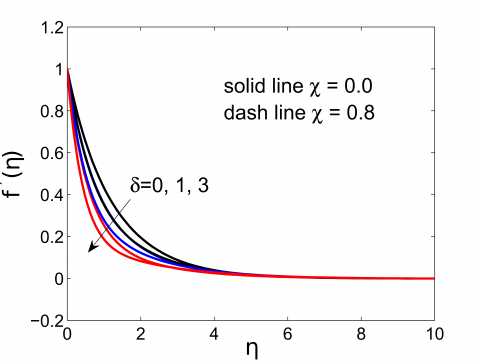(a) Porosity parameter effect on f'(η)

## 1b.png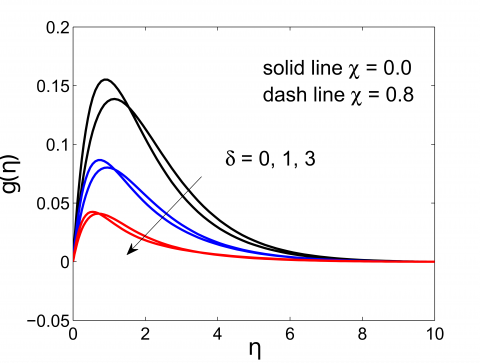(b) Porosity parameter effect on g(η)

## 1c.png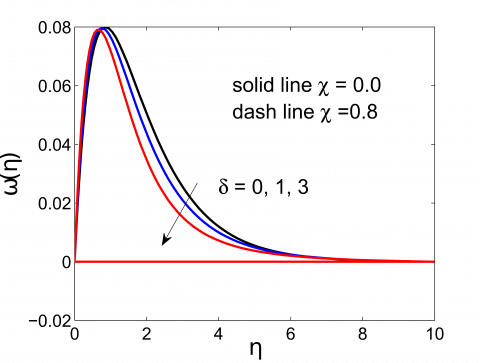(c) Porosity parameter effect on ω(η)

Figure 1. Primary, secondary velocities and microrotation profiles variation with δ

## 2a.png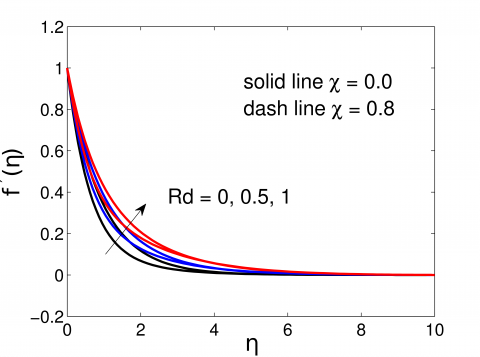(a) Radiation parameter effect on f'(η)

## 2b.png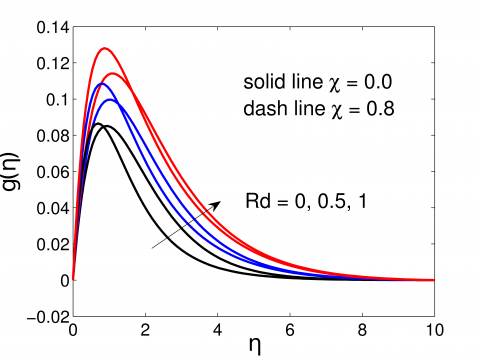(b) Radiation parameter effect on g(η)

## 2c.png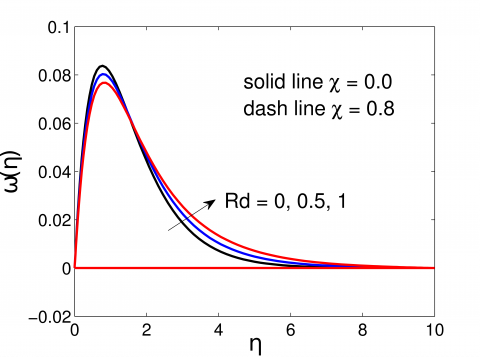(c) Radiation parameter effect on ω(η)

Figure 2. Primary, secondary velocities and microrotation profiles variation with Rd

The primary and secondary velocities increase with an increase in conduction radiation parameter can be seen in Figure 2(a) and Figure 2(c). This is because with the radiation parameter increase the absorption of radiated heat from the plate releases more heat energy to the fluid resulting buoyancy forces in the boundary layer increases fluid motion.

Physically, heat increases with radiation parameter increases and hence increase in both velocities. The microrotation show increase with increase around the boundary layer to its peak and then asymptotically flow to the downstream.

Figure 2(c) describes the microrotation profile for different values radiation parameter Rd. We observe a cross flow in the microrotation profile $\omega(\eta)$ at $\eta \cong 2$ in the reverse flow. The primary velocity and microrotation shows an increasing effect with hall parameter as seen in Figure 3(a) and Figure 3(b). When a magnetic field is applied perpendicular to the flow direction a reverse flow will be caused due to the force, Lorentz force, produced by the magnetic field. The flow caused due to this force is called the secondary flow. Reducing effect of magnetic field on the flow enhanced by Hall current parameter. Figure 3(b) shows the Hall effect on secondary velocity flow, when m=0 there is no flow in this component.

## 3a.png(a) Hall parameter effect on f'(η)

## 3b.png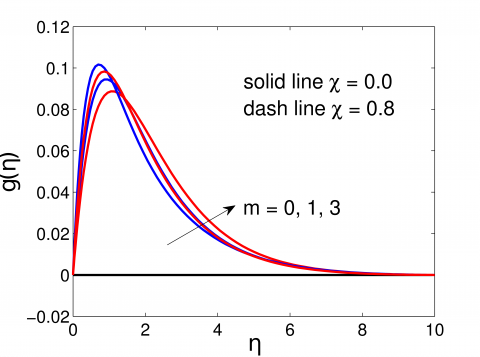(b) Radiation parameter effect on g(η)

## 3c.png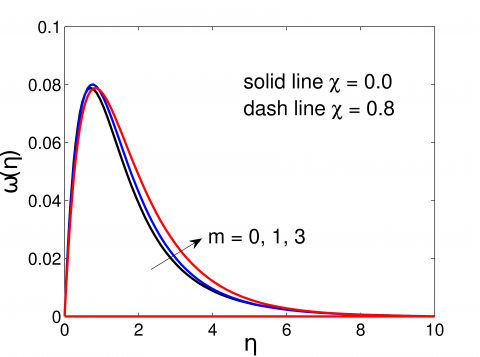(c) Radiation parameter effect on ω(η)

Figure 3. Primary, secondary velocities and microrotation profiles variation with m

## 4a.png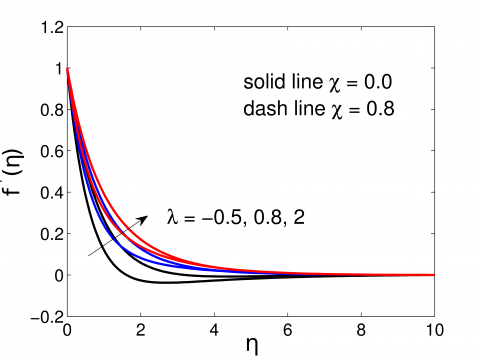(a) Mixed convection parameter effect on f'(η)

## 4b.png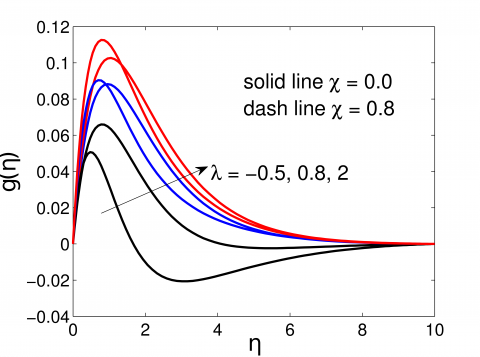(b) Mixed convection parameter effect on g(η)

## 4c.png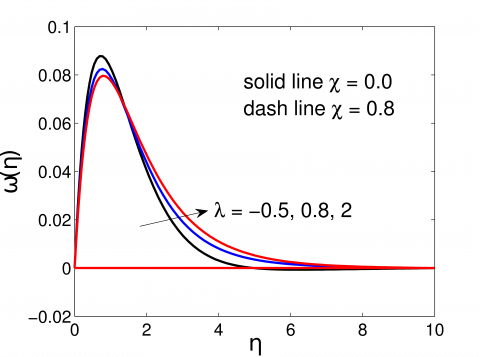(c) Mixed convection parameter effect on ω(η)

Figure 4. Primary, secondary velocities and microrotation profiles with λ

The mixed convection parameter effect is shown in Figure 4(b) indicates increasing in the secondary flow profile. It is interesting to see from Figure 4(b) for opposing flow λ<0 flows reversal.

4.2 Temperature, $\boldsymbol{\theta}$ profile

Figure 5 depicted the temperature profile varying the values of porosity parameter. Porosity parameters increase shows an increasing effect on the boundary layer. This is due to viscosity in $\frac{v_{f}}{k_{1}}$ in Eq. (2) and hence δ in Eq. (10) increase then friction in between the surface and fluid increases lead in temperature increase. In Figure 5(b) the decrease in secondary flow effect is more notable for viscus flow compared to micropolar fluid.

## 5a.png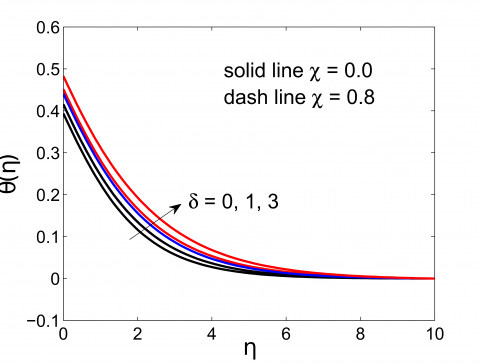(a) Porosity parameter effect on θ(η)

## 5b.png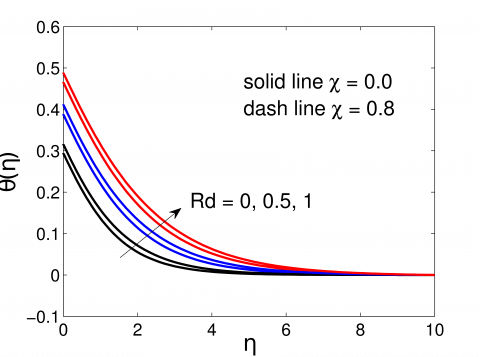(b) Radiation parameter effect on θ(η)

## 5c.png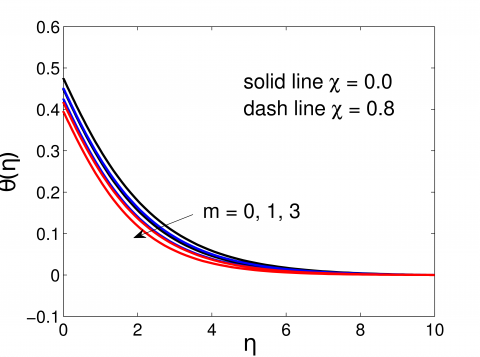(c) Hall parameter effect on θ(η)

## 5d.png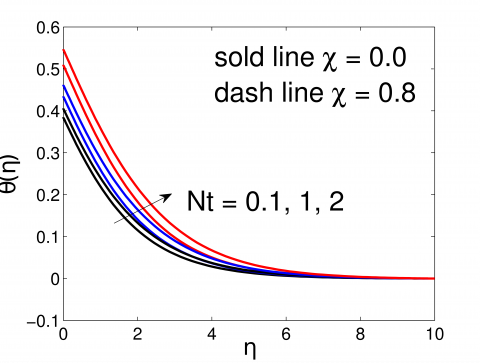(d) Thermophoresis parameter effect on θ(η)

## 5e.png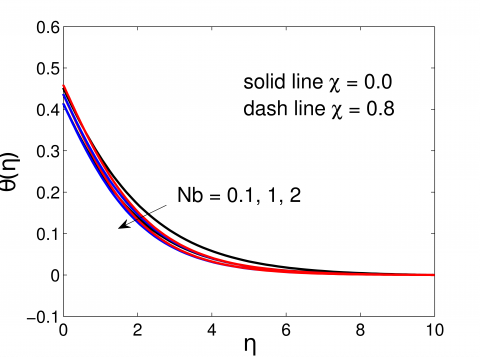(e) Brownian motion parameter effect on θ(η)

Figure 5. Temperature profiles

In Figure 5(b) increasing values of radiation parameter Rd show increase in temperature profile. Rosseland radiation absorption decrease when with more Rd which leads to more heat produced hence arise in temperature.

The effects of Hall parameter, m, on the temperature distributions is depicted in Figure 5(c). Generally, applied magnetic field decreases the temperature similar result is observed in the presence of nanoparticles also. Figure 5(d) shows increasing the thermophoresis parameter enhance the temperature. Physically the Brownian motion and thermophoresis parameters appeared due to presence of nanoparticles and the presence of these nanoparticles enhance thermal diffusion in the flow.

4.3 Nanoparticles concentration, φ profile

Figures 6(a) display the variations in dimensionless nanoparticles concentration profile for various values of porosity parameters. It demonstrates that nanoparticle concentration enhanced with increasing in porosity parameter. Figure 6(a) is plotted for the effect of porosity parameter. From Figure 1(a) we have seen velocity decreases with increase in porosity parameter, this increase in porosity parameter increases the concentration because of the nanoparticles in unit volume of the fluid enhance. Figure 6(b) depicts the effect of radiation parameter, Rd; shows increase the nanoparticles concentration profile. Figure 6(c) shows clear fall in nanoparticles concentration as Hall parameter increases. It is also noted that the viscous fluid enhances the flow than micropolar fluid. Figure 6(e) depicted the nanoparticle concentration profile for various values of thermophoresis parameter Nt. Since the nanoparticles related with the thermophoresis parameter results particle migration increase and momentum boundary layer decreases leads to more particles near the boundary layer region. The hot nanoparticles that are close to the plate are being pushed to the cold fluid at the ambient temperature due to thermophoresis force.

Table 1 presents the numerical outcomes of engineering parameters. Shear stresses decreases when Hall, radiation parameters and coupling number increases. Nusselt number and the density number of the motile microorganisms decrease with radiation and coupling parameters.

## 6a.png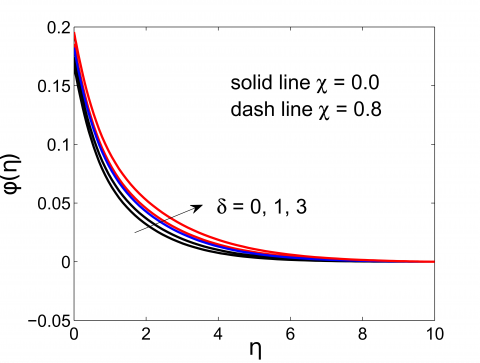(a) Porosity parameter effect on φ(η)

## 6b.png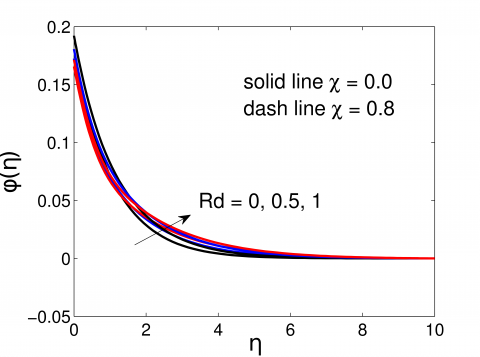(b) Radiation parameter effect on φ(η)

## 6c.png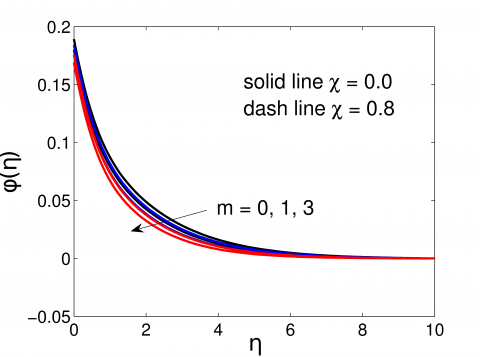(c) Hall parameter effect on φ(η)

## 6d.png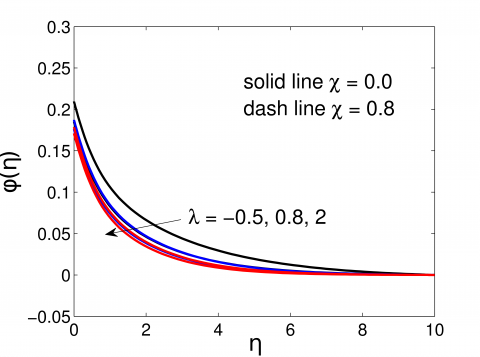(d) Mixed convection parameter effect on φ(η)

## 6e.png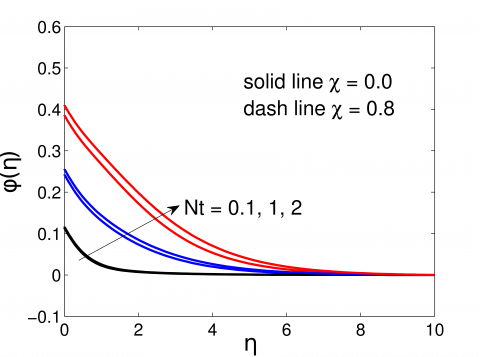(e) Thermophoresis parameter effect on φ(η)

Figure 6. Nanoparticles concentration profile

Table 1. Shearing stresses, the local Nusselt number, Sherwood number and the density number of the motile microorganisms. M=2, Nr=0.2, Rb=0.2, pr=1.0, Le=0.2, Lb=1.0, Nt=0.1, Nb=0.1, pe=2.0, β1=0.1, β2=0.1, β3=0.1, A=0.1, Ec=0.02, δ=0.5

 m Rd $\chi$ $-R e_{x}^{\frac{1}{2}}(1+\chi) \mathrm{Cf}_{\mathrm{x}}$ $R e_{x}^{-\frac{1}{2}} \mathrm{Nu}_{\mathrm{x}}$ $R e_{x}^{-\frac{1}{2}} S h_{\mathrm{x}}$ $R e_{x}^{-\frac{1}{2}} \mathrm{Nn}_{\mathrm{x}}$ 0.5 2 0.8 0.76399 0.07554 0.07127 0.09726 1 2 0.8 1.25610 0.18928 0.42385 0.88912 2 2 0.8 1.10915 0.19485 0.45519 0.91168 3 2 0.8 1.04765 0.19787 0.47030 0.92277 0.5 0 0.8 1.40812 0.12803 0.22287 0.03989 0.5 1 0.8 1.13217 0.19005 0.58564 0.90350 0.5 2 0.8 1.11888 0.19268 0.49947 0.90825 0.5 3 0.8 1.10915 0.19485 0.45519 0.91168 0.5 2 0 1.45902 0.17940 0.39461 0.84634 0.5 2 0.3 1.28630 0.18516 0.42049 0.87599 0.5 2 0.7 1.13803 0.19297 0.44909 0.90561 0.5 2 1 1.05802 0.19851 0.46627 0.92259

4.4 Gyrotactic microorganism concentration, h profile

The presence of radiation, porosity and magnetic force applied in the flow external stimuli the movement of microorganisms. Porosity parameter shows an increase in the gyrotactic microorganism profiles of the boundary layer thickness as can be seen in the Figure 7(a). Hall parameter decreases the Gyrotactic microorganisms concentration as seen in Figure 7(c). Figure 7(b) reports the effect of the radiation parameter, Rd, on Gyrotactic microorganisms concentration profile. Increasing the radiation parameter increases the Gyrotactic microorganisms concentration. Figure 7(e) depict as the Brownian motion parameter, Nb, increases the density of motile microorganisms decreases. This is because Nb will suppress the diffusion of nanoparticles away from the region.

## 7a.png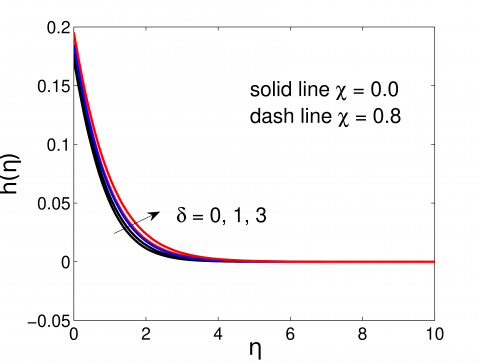(a) Porosity parameter effect on h(η)

## 7b.png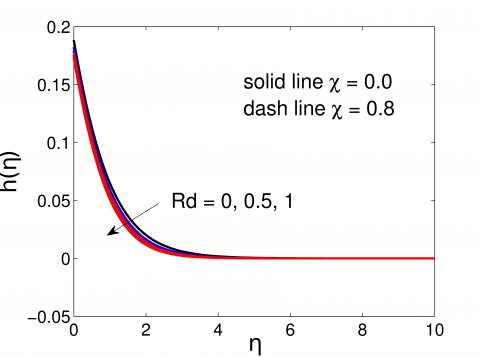(b) Radiation parameter effect on h(η)

## 7c.png(c) Hall parameter effect on h(η)

## 7d.png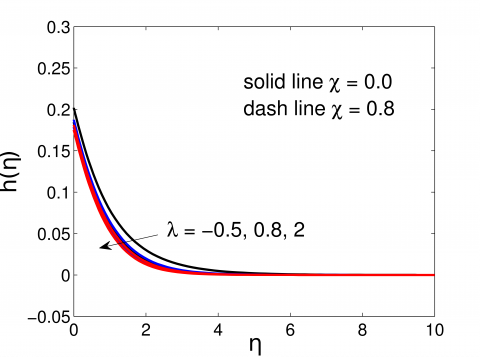(d) Mixed convection parameter effect on h(η)

## 7e.png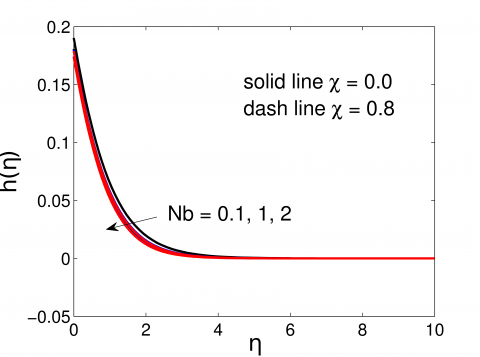(e) Thermophoresis parameter effect on h(η)

Figure 7. Gyrotactic microorganism concentration profile

5. Conclusion

Two-dimensional MHD steady incompressible flow, transfer of heat and mass in a micropolar nanofluid comprising gyrotactic microorganisms in porous medium is modelled. The numerical results were computed using the SQLM. The effects of various parameters for viscous and micropolar fluid studied and compared. The salient features are listed below:

• For the viscous flow, χ=0, the parameters $\delta$, Rd, m and λ show enhancing f', g and ω than micropolar fluid.

• Results disclose increase in the value of Hall current parameter leads to increase in the primary velocity and microrotation diminished for temperature nanoparticles concentration and gyrotactic microorganisms concentration.

• Greater porosity reduces velocities and microrotation whereas it enhances temperatures, nanoparticles concentration and gyrotactic microorganisms concentration.

• The microrotation and secondary velocity show a shoot to the pic and dwindle.

• An increment in λ and Rd corresponds to an enhancement in microrotation, the primary and secondary velocities.

• The local shear stress decreases with increase in the m, Rd and χ.

• Mass transfer increases with increase the Hall parameters.

Generally, the flow velocity when the fluid is micopolar shows a tendency of increase compared to the viscus fluid. On the other hand, micropolar fluid shows reduce in temperature profile than the viscous fluid.

Nomenclature
 A suction/injection parameter b chemotaxis constant (m) C Nanoparticles volume concentration g* acceleration due to gravity, (m s2) Lb bioconvection Lewis number Le Lewis number $\mathrm{f}(\eta)$ dimensionless stream function $\mathrm{h}(\mathrm{\eta})$ dimensionless number of motile microorganism j micro-inertia m Hall parameter M magnetic parameter N number density of motile microorganisms Nb Brownian motion parameter Nw wall motile microorganisms Nt Thermophoresis parameter Pe bioconvection Péclet number Pr Prandtl number Wc maximum cell swimming speed Rb Rayleigh number Rd Radiation parameter Greek symbols $\mathbf{V}_{\mathrm{f}}$ kinematic viscosity of fluid $\lambda$ Mixed convection parameter $\Gamma$ dimensional microrotation $\omega(\eta)$ Dimensionless microrotation $\varphi(\eta)$ dimensionless nanoparticles volume fraction $\rho f$ density of the fluid $\sigma$ electric conductivity $\sigma *$ Stefan-Boltzmann constant $\theta(\eta)$ nondimensional temperature Subscripts B0 constant magnetic field Cw Wall nanoparticles volume concentration $\mathrm{C}_{\infty}$ Ambient nanoparticles volume concentration DB Brownian diffusion coefficient (m2s-1) Dn microorganism diffusion coefficient (m2s-1) DT thermophoretic diffusion coefficient (m2s-1) hs heat transport (coefficient) hc mass transport (coefficient) hn heat transfer rate k1 Permeability of the porous medium kf thermal conductivity (wm-1 K-1) $\mu f$ Dynamic viscosity of the fluid (Pa s) ϗf vortex viscosity β1, β2, β3 constants qw constant wall heat flux (W m-1)
References

 Choi, S.U.S., Eastman, J.A. (1995). Enhancing thermal conductivity of fluids with nanoparticles (No. ANL/MSD/CP-84938; CONF-951135-29). Argonne National Lab., IL (United States).

 Buongiorno, J. (2006). Convective transport in nanofluids. Journal of Heat Transfer, 128(3): 240-250. https://doi.org/10.1115/1.2150834

 Nayak, M.K., Shaw, S., Makinde, O.D., Chamkha, A.J. (2018). Effects of homogenous–heterogeneous reactions on radiative NaCl-CNP nanofluid flow past a convectively heated vertical Riga plate. Journal of Nanofluids, 7(4): 657-667. https://doi.org/10.1166/jon.2018.1501

 Yadav, D., Wang, J. (2018). Convective heat transport in a heat generating porous layer saturated by a non-Newtonian nanofluid. Heat Transfer Engineering, 40(16): 1363-1382. https://doi.org/10.1080/01457632.2018.1470298

 Zahan, I., Alim, M.A. (2018). Effect of conjugate heat transfer on flow of nanofluid in a rectangular enclosure. International Journal of Heat and Technology, 36(2): 397-405. https://doi.org/10.18280/ijht.360203

 Mkhatshwa, M.P., Motsa, S.S., Ayano, M.S., Sibanda, P. (2020). MHD mixed convective nanofluid flow about a vertical slender cylinder using overlapping multi-domain spectral collocation approach. Case Studies in Thermal Engineering, 18: 100598. https://doi.org/10.1016/j.csite.2020.100598

 El Moutaouakil, L., Boukendil, M., Zrikem, Z., Abdelbaki, A. (2020). Natural convection and thermal radiation influence on nanofluids in a cubical cavity. International Journal of Heat and Technology, 38(1): 59-68. https://doi.org/10.18280/ijht.380107

 Sharma, B., Kumar, S., Paswan, M.K. (2018). Analytical solution for mixed convection and MHD flow of electrically conducting non-Newtonian nanofluid with different nanoparticles: A comparative study. International Journal of Heat and Technology, 36(3): 987-996. https://doi.org/10.18280/ijht.360327

 Tarakaramu, N., Satya Narayana, P.V. (2019). Chemical reaction effects on bio-convection nanofluid flow between two parallel plates in rotating system with variable viscosity: A numerical study. Journal of Applied and Computational Mechanics, 5(4): 791-803. https://doi.org/10.22055/JACM.2019.28147.1456

 Platt, J.R. (1961). "Bioconvection Patterns" in cultures of free-swimming organisms. Science, 133(3466): 1766-1767. https://doi.org/10.1126/science.133.3466.1766

 Khan, N.S., Gul, T., Khan, M.A., Bonyah, E., Islam, S. (2017). Mixed convection in gravity-driven thin film non-Newtonian nanofluids flow with gyrotactic microorganisms. Results in Physics, 7: 4033-4049. https://doi.org/10.1016/j.rinp.2017.10.017

 Waqas, H., Khan, S.U., Hassan, M., Bhatti, M.M., Imran, M. (2019). Analysis on the bioconvection flow of modified second-grade nanofluid containing gyrotactic microorganisms and nanoparticles. Journal of Molecular Liquids, 291: 111231. https://doi.org/10.1016/j.molliq.2019.111231

 Khan, S.U., Rauf, A., Shehzad, S.A., Abbas, Z., Javed, T. (2019). Study of bioconvection flow in Oldroyd-B nanofluid with motile organisms and effective Prandtl approach. Physica A: Statistical Mechanics and its Applications, 527: 121179. https://doi.org/10.1016/j.physa.2019.121179

 Zuhra, S., Khan, N.S., Shah, Z., Islam, S., Bonyah, E. (2018). Simulation of bioconvection in the suspension of second grade nanofluid containing nanoparticles and gyrotactic microorganisms. AIP Advances, 8(10): 105210. https://doi.org/10.1063/1.5054679

 Ramzan, M., Mohammad, M., Howari, F. (2019). Magnetized suspended carbon nanotubes based nanofluid flow with bio-convection and entropy generation past a vertical cone. Scientific Reports, 9(1): 1-15.

 Palwasha, Z., Islam, S., Khan, N.S., Ayaz, H. (2018). Non-Newtonian nanoliquids thin-film flow through a porous medium with magnetotactic microorganisms. Applied Nanoscience, 8(6): 1523-1544. https://doi.org/10.1007/s13204-018-0834-5

 Khan, N.S., Shah, Z., Islam, S., Khan, I., Alkanhal, T.A., Tlili, I. (2019). Entropy generation in MHD mixed convection non-Newtonian second-grade nanoliquid thin film flow through a porous medium with chemical reaction and stratification. Entropy, 21(2): 139.

  Mohsen, I., Seyed, A.M.M., Ali, J.C., Giulio, L. (2019). The impacts of heat generation/absorption and partial slip on boundary layer flow and heat transfer of a nanofluid comprising of self-impelled motile microorganisms passing a stretching sheet. Mathematical Modelling of Engineering Problems, 6(1): 10-20. https://doi.org/10.18280/mmep.060102

 Eringen, A.C. (1964). Simple microfluids. International Journal of Engineering Science, 2(2): 205-217. https://doi.org/10.1016/0020-7225(64)90005-9

 Ariman, T.M.A.N.D., Turk, M.A., Sylvester, N.D. (1973). Microcontinuum fluid mechanics—A review. International Journal of Engineering Science, 11(8): 905-930. https://doi.org/10.1016/0020-7225(73)90038-4

 Ariman, T.T.N.D., Turk, M.A., Sylvester, N.D. (1974). Applications of microcontinuum fluid mechanics. International Journal of Engineering Science, 12(4): 273-293. https://doi.org/10.1016/0020-7225(74)90059-7

 Balla, C.S., Alluguvelli, R., Naikoti, K., Makinde, O.D. (2020). Effect of chemical reaction on bioconvective flow in oxytactic microorganisms suspended porous cavity. Journal of Applied and Computational Mechanics, 6(3): 653-664. https://doi.org/10.22055/JACM.2019.14811

 Zuhra, S., Khan, N.S., Alam, M., Islam, S., Khan, A. (2020). Buoyancy effects on nanoliquids film flow through a porous medium with gyrotactic microorganisms and cubic autocatalysis chemical reaction. Advances in Mechanical Engineering, 12(1): 1687814019897510. https://doi.org/10.1177/1687814019897510

 Das, K., Duari, P.R., Kundu, P.K. (2015). Nanofluid bioconvection in presence of gyrotactic microorganisms and chemical reaction in a porous medium. Journal of Mechanical Science and Technology, 29(11): 4841-4849. https://doi.org/10.1007/s12206-015-1031-z

 Ahmed, S.E., Mahdy, A. (2016). Laminar MHD natural convection of nanofluid containing gyrotactic microorganisms over vertical wavy surface saturated non-Darcian porous media. Applied Mathematics and Mechanics, 37(4): 471-484. https://doi.org/10.1007%2Fs10483-016-2044-9

 Mekonnen, S.A., Olumuyiwa, O., Sicelo, P.G. (2019). Natural convection MHD radiative flow on a sphere through porous medium considering ohmic dissipation. International Journal of Heat and Technology, 37(4): 999-1008. https://doi.org/10.18280/ijht.370409

 Tripathy, R.S., Dash, G.C., Mishra, S.R., Hoque, M.M. (2016). Numerical analysis of hydromagnetic micropolar fluid along a stretching sheet embedded in porous medium with non-uniform heat source and chemical reaction. Engineering Science and Technology, an International Journal, 19(3): 1573-1581. https://doi.org/10.1016/j.jestch.2016.05.012

 Pop, I., Ingham, D.B. (2002). Transport Phenomena in Porous Media II. Elsevier.

 Nield, D.A., Bejan, A. (2006). Convection in Porous Media. 3rd edition, Springer, New York.

 Kuznetsov, A.V. (2011). Nanofluid bio-thermal convection: Simultaneous effects of gyrotactic and oxytactic micro-organisms. Fluid Dynamics Research, 43(5): 055505. https://doi.org/10.1088/0169-5983/43/5/055505

 Sarkar, A.K., Georgiou, G., Sharma, M.M. (1994). Transport of bacteria in porous media: I. An experimental investigation. Biotechnology and Bioengineering, 44(4): 489-497. https://doi.org/10.1002/bit.260440412

 Uddin, M.J., Kabir, M.N., Bég, O.A. (2016). Computational investigation of Stefan blowing and multiple-slip effects on buoyancy-driven bioconvection nanofluid flow with microorganisms. International Journal of Heat and Mass Transfer, 95: 116-130. https://doi.org/10.1016/j.ijheatmasstransfer.2015.11.015

 Bellman, R.E., Kalaba, R.E. (1965). Quasilinearisation and Non-Linear Boundary-Value Problems. Elsevier, New York.

 Motsa, S.S., Sibanda, P., Shateyi, S. (2011). On a new quasi‐linearization method for systems of nonlinear boundary value problems. Mathematical Methods in the Applied Sciences, 34(11): 1406-1413. https://doi.org/10.1002/mma.1449

 Trefethen, L.N. (2000). Spectral methods in MATLAB. Society for Industrial and Applied Mathematics.

 Hussaini, M.Y., Zang, T.A. (1987). Spectral methods in fluid dynamics. Annual Review of Fluid Mechanics, 19(1): 339-367. https://doi.org/10.1146/annurev.fl.19.010187.002011